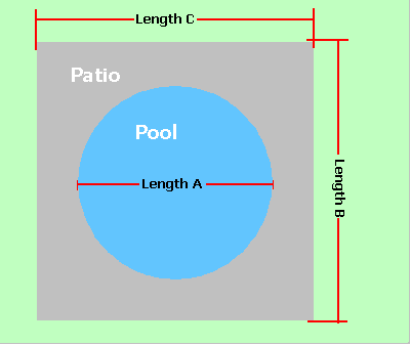Spike's Calculators

# Square Rectangular Shaped Concrete Patio - Round Pool - Metric Measurements

Calculate the amount of concrete needed to pour a rectangular/square shaped slab around a round pool, using Metric measurements for the calculations.

Included in the results, the square metres of the patio area and the outer perimeter to be able to calculate for use of different materials and to estimate the materials for a fence.### Square/Rectangular Slab - Round Pool

Diameter of Pool Length A m
Length of Outer Area Length B m
Length of Outer Area Length C m
Required Slab Depth mm

#### Results:

 Area of Pool m² Area of Pool Patio m² Outer Perimeter lin m Concrete Needed in Cubic Metres m³ Concrete Needed in Cubic Yards yd³

#### Calculations

1. the diameter of the pool (length A in metres)
2. the length of the overall area (length B in metres)
3. the width of the overall area (length C in metres)
4. depth of concrete slab (millimetres)

#### Results

1. square metres of the pool area
2. square metres of the patio area around the pool
3. outer perimeter of the patio area (lineal metres)
4. concrete needed in cubic metres
5. concrete needed in cubic yards